# Skew-field

(diff) ← Older revision | Latest revision (diff) | Newer revision → (diff)
A ring in which the equations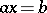and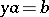with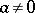are uniquely solvable. In the case of an associative ring (cf. Associative rings and algebras) it is sufficient to require the existence of a unit 1 and the unique solvability of the equations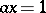and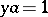for any. A commutative associative skew-field is called a field. An example of a non-commutative associative skew-field is the skew-field of quaternions, defined as the set of matrices of the form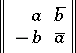over the field of complex numbers with the usual operations (see Quaternion). An example of a non-associative skew-field is the Cayley–Dickson algebra, consisting of all matrices of the same form as above over the skew-field of quaternions. This skew-field is alternative (see Alternative rings and algebras). Any skew-field is a division algebra either over the field of rational numbers or over a field of residues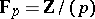. The skew-field of quaternions is a-dimensional algebra over the field of real numbers, while the Cayley–Dickson algebra is-dimensional. The dimension of any algebra with division over the field of real numbers is equal to 1, 2, 4, or 8 (see , and also Topological ring). The fields of real or complex numbers and the skew-field of quaternions are the only connected locally compact associative skew-fields (see ). Any finite-dimensional algebra without zero divisors is a skew-field. Any finite associative skew-field is commutative (see , ). An associative skew-field is characterized by the property that any non-zero module over it is free. Any non-associative skew-field is finite-dimensional . A similar result applies to Mal'tsev skew-fields  (see Mal'tsev algebra) and to Jordan skew-fields  (see Jordan algebra). In contrast to the commutative case, not every associative ring without zero divisors can be imbedded in a skew-field (see Imbedding of rings).